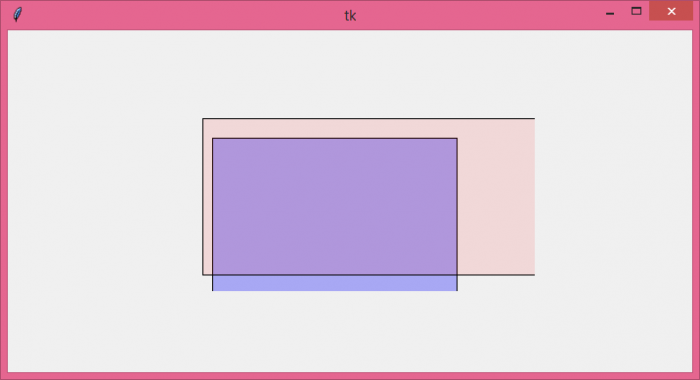# How to make a Tkinter canvas rectangle transparent?

The canvas widget is one of the most versatile widgets in Tkinter Library. Generally, it is used to draw shapes, animate objects, and create complex graphics in any application. To create shapes like Rectangle, we use the create_rectangle(x,y, x+ width, y+ height, **options) method. We can configure the item on the canvas by adding properties such as width, height, fill and bg, border width, etc.

The alpha property in canvas defines the transparency of the canvas item. However, the property is not available in the Tkinter library; thus, we must define a function to provide the transparency attribute in shape. The steps to create the function for transparency attribute are,

• Define an inbuilt function create_rectangle(x,y,a,b, **options).
• Calculate the alpha for each color (RGB) that must be provided to the shape.
• Remove the predefined alpha (if applicable) from the shape using pop().
• Calculate the shape color in the region using winfo_rgb() and add the alpha to the shape.
• Since a newly created shape will have different color and background, thus it must be needed to use this as an image.
• The Image can be displayed in the canvas easily.

## Example

# Import the required libraries
from tkinter import *
from PIL import Image, ImageTk

# Create an instance of tkinter frame
win= Tk()

# Set the size of the tkinter window
win.geometry("700x350")

# Store newly created image
images=[]

# Define a function to make the transparent rectangle
def create_rectangle(x,y,a,b,**options):
if 'alpha' in options:
# Calculate the alpha transparency for every color(RGB)
alpha = int(options.pop('alpha') * 255)
# Use the fill variable to fill the shape with transparent color
fill = options.pop('fill')
fill = win.winfo_rgb(fill) + (alpha,)
image = Image.new('RGBA', (a-x, b-y), fill)
images.append(ImageTk.PhotoImage(image))
canvas.create_image(x, y, image=images[-1], anchor='nw')
canvas.create_rectangle(x, y,a,b, **options)
canvas= Canvas(win)

# Create a rectangle in canvas
create_rectangle(50, 110,300,280, fill= "blue", alpha=.3)
create_rectangle(40, 90, 420, 250, fill= "red", alpha= .1)
canvas.pack()

win.mainloop()

## Output

Running the above code will display multiple transparent rectangles in the canvas.